# 晴空湍流对飞机的影响分析Analysis of Influence of Clear Air Turbulence on Aircraft

• 全文下载: PDF(856KB)    PP.1-10   DOI: 10.12677/OJTT.2019.81001
• 下载量: 192  浏览量: 367   科研立项经费支持

It is very difficult for civil aviation aircraft to predict CAT (clear air turbulence), since the precipitation of CAT is lower than that of the convective turbulence. So CAT is a major hidden danger affecting aviation safety. In order to detect CAT as effectively as possible, firstly, the influence of CAT on the aircraft is analyzed in this paper, and a reactive CAT detection method is researched. This method is estimating the Eddy Dissipation Rate (EDR) based on the aircraft's vertical acceleration to quantify the CAT intensity. Then, the detection process is obtained. Secondly, The Radio Technical Commission for Aeronautics revised the specification of airborne weather radar detection turbulence, in which the vertical load factor is defined as the detection amount. The relationship between the EDR and the vertical load factor can be further analyzed in detail to conclude that the impact of the CAT on the aircraft can be quantified as the vertical load factor. Finally, the conclusion of the above theoretical analysis is verified to be correct, as the EDR is calculated using the real ADS-B (Automatic Dependent Surveillance-Broadcast) data which come from a flight that has encountered CAT. Furthermore, it is proved that the CAT detection method based on the vertical load factor is reasonable, and airborne weather radars can predict CAT more accurately and reasonably by using this method. The analysis of the impact of clear-air turbulence on the aircraft has practical significance for the accurate prediction and detection of clear-air turbulence.

1. 引言

2. 基于飞机垂直加速度的EDR估计

2.1. 基本思想

$\epsilon =B{\sigma }_{T}^{3}{V}^{-1}$ (1)

2.2. 飞机响应因子

$B$ 是特定飞机的响应因子，取决于飞机特性(机翼面积，飞机质量等)和飞行条件(真空速，飞行高度等)  。

${H}_{rg}\left(\omega \right)=\frac{\stackrel{¨}{z}}{{w}_{g0}}=-{\omega }^{2}\frac{-\frac{1}{2}\rho V{S}_{W}a}{-{\omega }^{2}m+i\omega \frac{1}{2}\rho V{S}_{W}a}$ (2)

$B=1/\left[{0.7}^{3/2}{I}^{3/2}\right]$ (3)

$I=\int {|{H}_{\text{r}g}\left(\omega \right)|}^{2}{\omega }^{-5/3}\text{d}\omega$ (4)

2.3. 基于ADS-B数据估计 ${\sigma }_{T}$

$\frac{\text{d}z}{\text{d}t}={v}_{z}$ (5)

$\frac{\text{d}{v}_{z}}{\text{d}t}={a}_{z}$ (6)Table 1. Categorization of turbulence intensity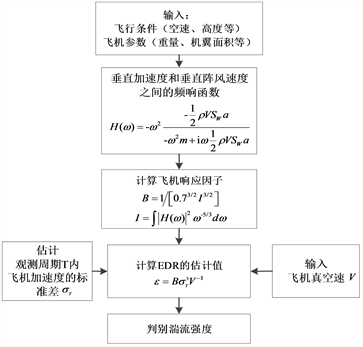Figure 1. Calculation flowchart of EDR based on aircraft vertical acceleration

3. CAT对飞机的影响量化

3.1. 基于垂直载荷因子的晴空湍流检测方法的基本思想

${\stackrel{^}{\sigma }}_{\Delta n}=\frac{{\sigma }_{\Delta n}}{unit{\sigma }_{w}}\frac{{\left[{\stackrel{¯}{M}}_{2}\left(x\right)\right]}^{0.5}}{\frac{\sqrt{{\sigma }_{v}^{2}\left(r\right)}}{\sigma }}$ (7)

3.2. 垂直载荷因子与EDR的关系

4. 验证和分析Figure 2. The actual flight altitude and ground speed of the aircraftFigure 3. Partial enlarged view of the flight altitude of the aircraft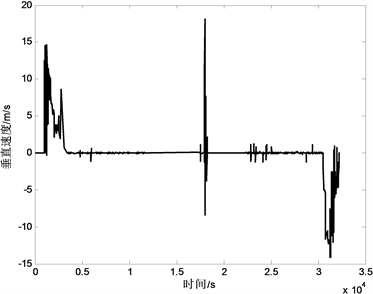Figure 4. The vertical speed of the aircraft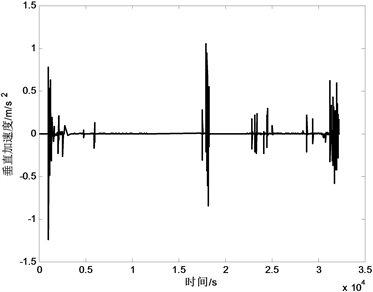Figure 5. The vertical acceleration of the aircraft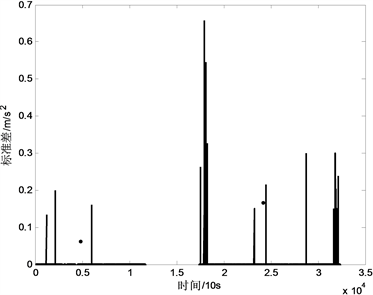Figure 6. Standard deviation of vertical acceleration of the aircraft, T = 10Figure 7. The power density function of ${|H\left(\omega \right)|}^{2}{\omega }^{-5/3}$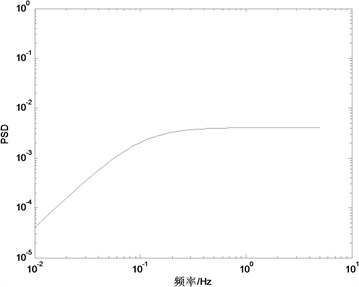Figure 8. The power density function of ${|H\left(\omega \right)|}^{2}$

5. 结论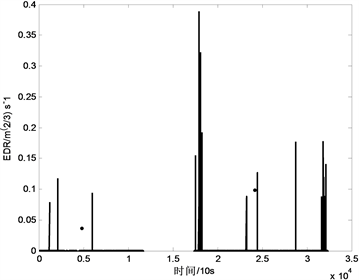Figure 9. EDR estimate, T = 10 s

  Klimenko, V. and Krozel, J. (2011) Clear-Air Turbulence Impact Modeling Based on Flight Route Analysis. AIAA Guidance, Navigation, and Control Conference, Portland, 8-11 August 2011, 6513. https://doi.org/10.2514/6.2011-6513  周林, 黄超凡. 近10年晴空湍流的研究进展[J]. 气象科技, 2015, 43(1): 91-96.  云新网. A330客机遭遇魔鬼湍流, 机舱秒变拆房现场, 15人受伤[Z/OL]. https://www.yn199.cn/112810-1.html, 2018-10-22.  Ejinsight (2017) Nine Injured after KLM Flight Hits Turbulence En Route to HK. http://www.ejinsight.com/20170605-nine-injured-after-klm-flight-hits-turbulence-en-route-to-hk/  Williams, P.D. (2017) Increased Light, Moderate, and Severe Clear-Air Turbulence in Response to Climate Change. Advances in Atmospheric Sciences, 34, 576-586. https://doi.org/10.1007/s00376-017-6268-2  Takacs, A., et al. (2006) Using In Situ Eddy Dissipation Rate (EDR) Observations for Turbulence Forecast Verification. 12th Conference on Aviation Range and Aerospace Meteorology, Atlanta, 31 January 2006.  Painting, D.J. (2003) Aircraft Meteorological Data Relay (AMDAR) Reference Manual. World Meteorological Organization Doc WMO-958.  Kopeć, J.M., Kwiatkowski, K., de Haan, S., et al. (2015) Retrieving Clear-Air Turbulence Information from Regular Commercial Aircraft Using Mode-S and ADS-B Broadcast. Atmospheric Measurement Techniques Discussions, 8, 11817-11852. https://doi.org/10.5194/amtd-8-11817-2015  卢晓光, 夏冬. 基于统计置信度的湍流检测门限确定方法[J]. 中国民航大学学报, 2011, 29(4): 27-30.  RTCA, Inc. (2016) RTCA/DO-220A Minimum Operational Performance Standards (MOPS) for Airborne Weather Radar System.  Li, Q., Rapp, M., Schrön, A., et al. (2016) Derivation of Turbulent Energy Dissipation Rate with the Middle Atmosphere Alomar Radar System (MAARSY) and Radiosondes at Andøya, Norway. Annales Geophysicae, 34, 1209. https://doi.org/10.5194/angeo-34-1209-2016  Wright, J.R. and Cooper, J.E. (2008) Introduction to Aircraft Aeroelasticity and Loads. John Wiley & Sons, Hoboken.  Bowles, R.L. and Buck, B.K. (2009) A Methodology for Determining Statistical Performance Compliance for Airborne Doppler Radar with Forward-Looking Turbulence Detection Capability. NASA CR, 215769.